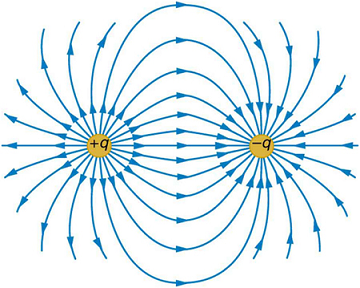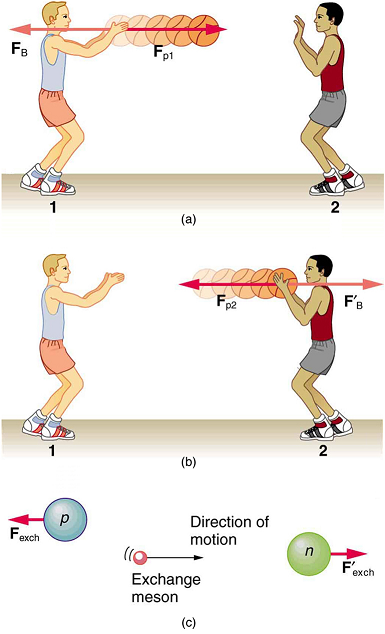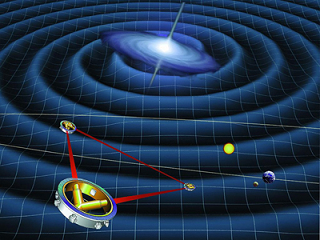$$\require{cancel}$$

# 4.8: Extended Topic: The Four Basic Forces—An Introduction

•• Contributed by OpenStax
• General Physics at OpenStax CNX

One of the most remarkable simplifications in physics is that only four distinct forces account for all known phenomena. In fact, nearly all of the forces we experience directly are due to only one basic force, called the electromagnetic force. (The gravitational force is the only force we experience directly that is not electromagnetic.) This is a tremendous simplification of the myriad of apparently different forces we can list, only a few of which were discussed in the previous section. As we will see, the basic forces are all thought to act through the exchange of microscopic carrier particles, and the characteristics of the basic forces are determined by the types of particles exchanged. Action at a distance, such as the gravitational force of Earth on the Moon, is explained by the existence of a force field rather than by “physical contact.”

The four basic forces are the gravitational force, the electromagnetic force, the weak nuclear force, and the strong nuclear force. Their properties are summarized in Table. Since the weak and strong nuclear forces act over an extremely short range, the size of a nucleus or less, we do not experience them directly, although they are crucial to the very structure of matter. These forces determine which nuclei are stable and which decay, and they are the basis of the release of energy in certain nuclear reactions. Nuclear forces determine not only the stability of nuclei, but also the relative abundance of elements in nature. The properties of the nucleus of an atom determine the number of electrons it has and, thus, indirectly determine the chemistry of the atom. More will be said of all of these topics in later chapters.

CONCEPT CONNECTIONS: THE FOUR BASIC FORCES

The four basic forces will be encountered in more detail as you progress through the text. The gravitational force is defined in Uniform Circular Motion and Gravitation, electric force in Electric Charge and Electric Field, magnetic force in Magnetism, and nuclear forces in Radioactivity and Nuclear Physics. On a macroscopic scale, electromagnetism and gravity are the basis for all forces. The nuclear forces are vital to the substructure of matter, but they are not directly experienced on the macroscopic scale.

Force Approximate relative strengths Range Attraction/Repulsion Carrier Particle
Gravitational $$10^{-38}$$ $$\infty$$ attractive only Graviton
Electromagnetic $$10^{-2}$$ $$\infty$$ attractive and repulsive Photon
Weak Nuclear $$10^{-13}$$ $$<10^{-18} \, m$$ attractive and repulsive $$W^+, \, W^-, \, Z^0$$
Strong Nuclear $$1$$ $$<10^{-15} \, m$$ attractive and repulsive gluons

The gravitational force is surprisingly weak—it is only because gravity is always attractive that we notice it at all. Our weight is the gravitational force due to the entire Earth acting on us. On the very large scale, as in astronomical systems, the gravitational force is the dominant force determining the motions of moons, planets, stars, and galaxies. The gravitational force also affects the nature of space and time. As we shall see later in the study of general relativity, space is curved in the vicinity of very massive bodies, such as the Sun, and time actually slows down near massive bodies.

Electromagnetic forces can be either attractive or repulsive. They are long-range forces, which act over extremely large distances, and they nearly cancel for macroscopic objects. (Remember that it is the net external force that is important.) If they did not cancel, electromagnetic forces would completely overwhelm the gravitational force. The electromagnetic force is a combination of electrical forces (such as those that cause static electricity) and magnetic forces (such as those that affect a compass needle). These two forces were thought to be quite distinct until early in the 19th century, when scientists began to discover that they are different manifestations of the same force. This discovery is a classical case of the unification of forces. Similarly, friction, tension, and all of the other classes of forces we experience directly (except gravity, of course) are due to electromagnetic interactions of atoms and molecules. It is still convenient to consider these forces separately in specific applications, however, because of the ways they manifest themselves.

CONCEPT CONNECTIONS: UNIFYING FORCES

Attempts to unify the four basic forces are discussed in relation to elementary particles later in this text. By “unify” we mean finding connections between the forces that show that they are different manifestations of a single force. Even if such unification is achieved, the forces will retain their separate characteristics on the macroscopic scale and may be identical only under extreme conditions such as those existing in the early universe.

Physicists are now exploring whether the four basic forces are in some way related. Attempts to unify all forces into one come under the rubric of Grand Unified Theories (GUTs), with which there has been some success in recent years. It is now known that under conditions of extremely high density and temperature, such as existed in the early universe, the electromagnetic and weak nuclear forces are indistinguishable. They can now be considered to be different manifestations of one force, called the electroweak force. So the list of four has been reduced in a sense to only three. Further progress in unifying all forces is proving difficult—especially the inclusion of the gravitational force, which has the special characteristics of affecting the space and time in which the other forces exist.

While the unification of forces will not affect how we discuss forces in this text, it is fascinating that such underlying simplicity exists in the face of the overt complexity of the universe. There is no reason that nature must be simple—it simply is.

# Action at a Distance: Concept of a Field

All forces act at a distance. This is obvious for the gravitational force. Earth and the Moon, for example, interact without coming into contact. It is also true for all other forces. Friction, for example, is an electromagnetic force between atoms that may not actually touch. What is it that carries forces between objects? One way to answer this question is to imagine that a force field surrounds whatever object creates the force. A second object (often called a test object) placed in this field will experience a force that is a function of location and other variables. The field itself is the “thing” that carries the force from one object to another. The field is defined so as to be a characteristic of the object creating it; the field does not depend on the test object placed in it. Earth’s gravitational field, for example, is a function of the mass of Earth and the distance from its center, independent of the presence of other masses. The concept of a field is useful because equations can be written for force fields surrounding objects (for gravity, this yields $$w = mg$$ at Earth’s surface), and motions can be calculated from these equations. (See Figure.)Figure $$\PageIndex{1}$$: The electric force field between a positively charged particle and a negatively charged particle. When a positive test charge is placed in the field, the charge will experience a force in the direction of the force field lines.

CONCEPT CONNECTIONS: FORCE FIELDS

The concept of a force field is also used in connection with electric charge and is presented in Electric Charge and Electric Field. It is also a useful idea for all the basic forces, as will be seen in Particle Physics. Fields help us to visualize forces and how they are transmitted, as well as to describe them with precision and to link forces with subatomic carrier particles.

The field concept has been applied very successfully; we can calculate motions and describe nature to high precision using field equations. As useful as the field concept is, however, it leaves unanswered the question of what carries the force. It has been proposed in recent decades, starting in 1935 with Hideki Yukawa’s (1907–1981) work on the strong nuclear force, that all forces are transmitted by the exchange of elementary particles. We can visualize particle exchange as analogous to macroscopic phenomena such as two people passing a basketball back and forth, thereby exerting a repulsive force without touching one another. (See Figure.)Figure $$\PageIndex{2}$$:The exchange of masses resulting in repulsive forces. (a) The person throwing the basketball exerts a force $$F_{p1}$$ on it toward the other person and feels a reaction force $$F_B$$ away from the second person. (b) The person catching the basketball exerts a force$$F_{p2}$$ on it to stop the ball and feels a reaction force $$F'_B$$ away from the first person. (c) The analogous exchange of a meson between a proton and a neutron carries the strong nuclear forces $$F_{exch}$$ and $$F'_{exch}$$ between them. An attractive force can also be exerted by the exchange of a mass—if person 2 pulled the basketball away from the first person as he tried to retain it, then the force between them would be attractive

This idea of particle exchange deepens rather than contradicts field concepts. It is more satisfying philosophically to think of something physical actually moving between objects acting at a distance. Table lists the exchange or carrier particles, both observed and proposed, that carry the four forces. But the real fruit of the particle-exchange proposal is that searches for Yukawa’s proposed particle found it and a number of others that were completely unexpected, stimulating yet more research. All of this research eventually led to the proposal of quarks as the underlying substructure of matter, which is a basic tenet of GUTs. If successful, these theories will explain not only forces, but also the structure of matter itself. Yet physics is an experimental science, so the test of these theories must lie in the domain of the real world. As of this writing, scientists at the CERN laboratory in Switzerland are starting to test these theories using the world’s largest particle accelerator: the Large Hadron Collider. This accelerator (27 km in circumference) allows two high-energy proton beams, traveling in opposite directions, to collide. An energy of 14 trillion electron volts will be available. It is anticipated that some new particles, possibly force carrier particles, will be found. (See Figure.) One of the force carriers of high interest that researchers hope to detect is the Higgs boson. The observation of its properties might tell us why different particles have different masses.Figure $$\PageIndex{3}$$:The world’s largest particle accelerator spans the border between Switzerland and France. Two beams, traveling in opposite directions close to the speed of light, collide in a tube similar to the central tube shown here. External magnets determine the beam’s path. Special detectors will analyze particles created in these collisions. Questions as broad as what is the origin of mass and what was matter like the first few seconds of our universe will be explored. This accelerator began preliminary operation in 2008. (credit: Frank Hommes)

Tiny particles also have wave-like behavior, something we will explore more in a later chapter. To better understand force-carrier particles from another perspective, let us consider gravity. The search for gravitational waves has been going on for a number of years. Almost 100 years ago, Einstein predicted the existence of these waves as part of his general theory of relativity. Gravitational waves are created during the collision of massive stars, in black holes, or in supernova explosions—like shock waves. These gravitational waves will travel through space from such sites much like a pebble dropped into a pond sends out ripples—except these waves move at the speed of light. A detector apparatus has been built in the U.S., consisting of two large installations nearly 3000 km apart—one in Washington state and one in Louisiana! The facility is called the Laser Interferometer Gravitational-Wave Observatory (LIGO). Each installation is designed to use optical lasers to examine any slight shift in the relative positions of two masses due to the effect of gravity waves. The two sites allow simultaneous measurements of these small effects to be separated from other natural phenomena, such as earthquakes. Initial operation of the detectors began in 2002, and work is proceeding on increasing their sensitivity. Similar installations have been built in Italy (VIRGO), Germany (GEO600), and Japan (TAMA300) to provide a worldwide network of gravitational wave detectors.

International collaboration in this area is moving into space with the joint EU/US project LISA (Laser Interferometer Space Antenna). Earthquakes and other Earthly noises will be no problem for these monitoring spacecraft. LISA will complement LIGO by looking at much more massive black holes through the observation of gravitational-wave sources emitting much larger wavelengths. Three satellites will be placed in space above Earth in an equilateral triangle (with 5,000,000-km sides) (Figure). The system will measure the relative positions of each satellite to detect passing gravitational waves. Accuracy to within 10% of the size of an atom will be needed to detect any waves. The launch of this project might be as early as 2018.

“I’m sure LIGO will tell us something about the universe that we didn’t know before. The history of science tells us that any time you go where you haven’t been before, you usually find something that really shakes the scientific paradigms of the day. Whether gravitational wave astrophysics will do that, only time will tell.” —David Reitze, LIGO Input Optics Manager, University of Florida.Figure $$\PageIndex{4}$$:Space-based future experiments for the measurement of gravitational waves. Shown here is a drawing of LISA’s orbit. Each satellite of LISA will consist of a laser source and a mass. The lasers will transmit a signal to measure the distance between each satellite’s test mass. The relative motion of these masses will provide information about passing gravitational waves. (credit: NASA)

The ideas presented in this section are but a glimpse into topics of modern physics that will be covered in much greater depth in later chapters.

# Summary

• The various types of forces that are categorized for use in many applications are all manifestations of the four basic forces in nature.
• The properties of these forces are summarized in Table.
• Everything we experience directly without sensitive instruments is due to either electromagnetic forces or gravitational forces. The nuclear forces are responsible for the submicroscopic structure of matter, but they are not directly sensed because of their short ranges. Attempts are being made to show all four forces are different manifestations of a single unified force.
• A force field surrounds an object creating a force and is the carrier of that force.

## Footnotes

The graviton is a proposed particle, though it has not yet been observed by scientists. See the discussion of gravitational waves later in this section. The particles $$W^+, \, W^-,$$ and $$Z^0$$ are called vector bosons; these were predicted by theory and first observed in 1983. There are eight types of gluons proposed by scientists, and their existence is indicated by meson exchange in the nuclei of atoms.

## Glossary

carrier particle
a fundamental particle of nature that is surrounded by a characteristic force field; photons are carrier particles of the electromagnetic force
force field
a region in which a test particle will experience a force

## Contributors

Paul Peter Urone (Professor Emeritus at California State University, Sacramento) and Roger Hinrichs (State University of New York, College at Oswego) with Contributing Authors: Kim Dirks (University of Auckland) and Manjula Sharma (University of Sydney). This work is licensed by OpenStax University Physics under a Creative Commons Attribution License (by 4.0).